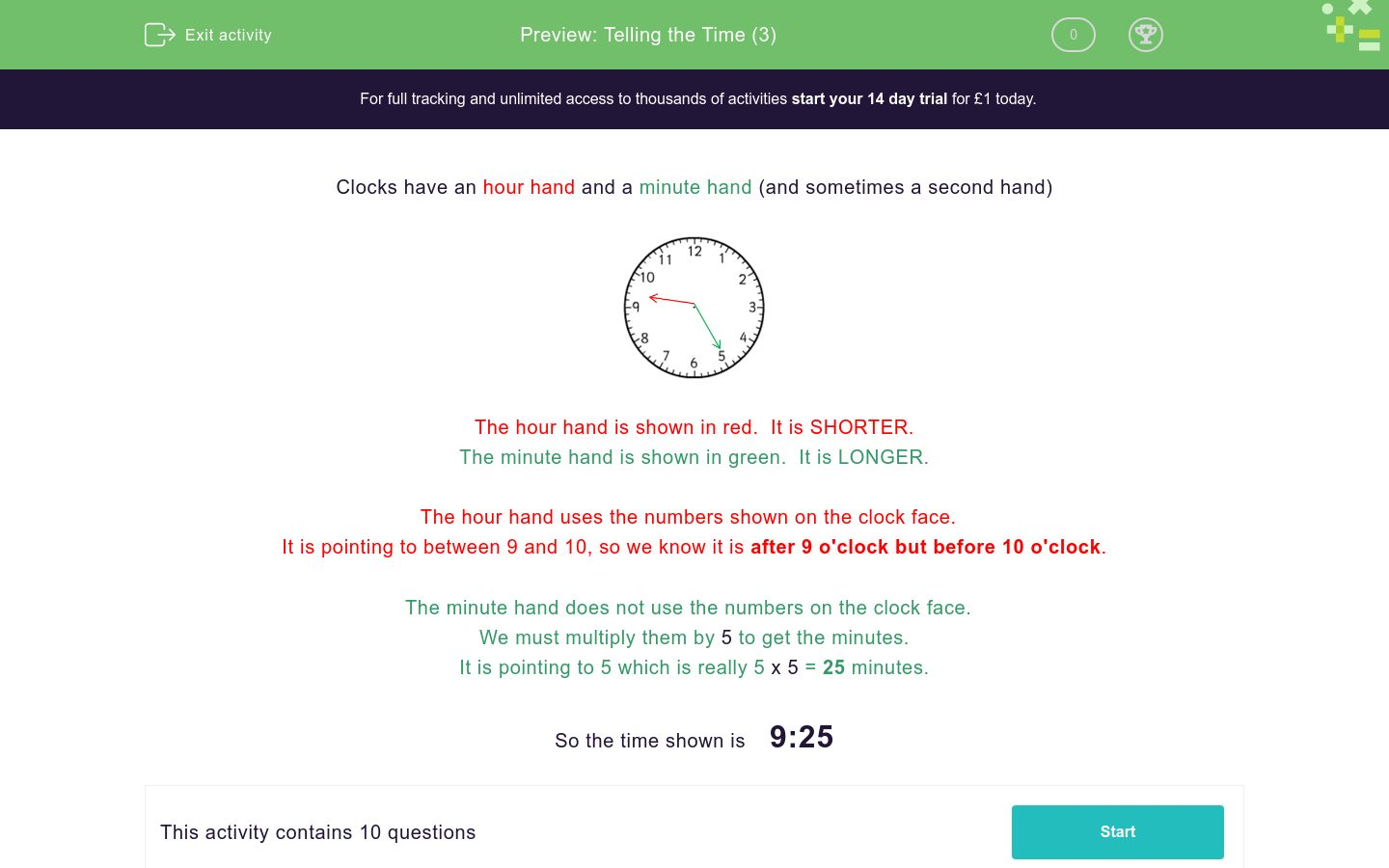# Telling the Time (3)

In this worksheet, students tell the time from a standard clock face.Key stage:  KS 1

Curriculum topic:  Measurement

Curriculum subtopic:  Tell/Write the Time

Difficulty level:### QUESTION 1 of 10

Clocks have an hour hand and a minute hand (and sometimes a second hand)The hour hand is shown in red.  It is SHORTER.

The minute hand is shown in green.  It is LONGER.

The hour hand uses the numbers shown on the clock face.

It is pointing to between 9 and 10, so we know it is after 9 o'clock but before 10 o'clock.

The minute hand does not use the numbers on the clock face.

We must multiply them by 5 to get the minutes.

It is pointing to 5 which is really 5 x 5 = 25 minutes.

So the time shown is    9:25

Match each clockface to the correct time.## Column B

A
1:50
B
1:55
C
10:10

Match each clockface to the correct time.## Column B

A
8:40
B
7:40
C
7:35

Match each clockface to the correct time.## Column B

A
5:55
B
10:25
C
6:50
Not Shown
10:30

To which number on the clockface does the hour hand point at 2 o'clock ?

To which number on the clockface does the minute hand point at 2 o'clock ?

To which number on the clockface does the minute hand point at 2:25 ?

What is the time ten minutes after the time shown on the clockface.4:45

4:11

4:55

What was the time ten minutes before the time shown on the clockface.4:35

4:07

4:55

What is the time half an hour before the time shown on the clockface.4:35

4:15

5:15

What is the time half an hour after the time shown on the clockface.4:15

5:15

9:55

• Question 1

Match each clockface to the correct time.## Column B

A
1:55
B
10:10
C
1:50
• Question 2

Match each clockface to the correct time.## Column B

A
7:40
B
7:35
C
8:40
• Question 3

Match each clockface to the correct time.## Column B

A
5:55
B
10:25
C
10:30
Not Shown
6:50
• Question 4

To which number on the clockface does the hour hand point at 2 o'clock ?

2
• Question 5

To which number on the clockface does the minute hand point at 2 o'clock ?

12
• Question 6

To which number on the clockface does the minute hand point at 2:25 ?

5
• Question 7

What is the time ten minutes after the time shown on the clockface.4:55
• Question 8

What was the time ten minutes before the time shown on the clockface.4:35
• Question 9

What is the time half an hour before the time shown on the clockface.4:15
• Question 10

What is the time half an hour after the time shown on the clockface.